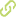A proposed curvilinearity index for quantifying airflow obstruction Journal ArticleOverview
abstract
• BACKGROUND: Though forced expiratory volume in the first second (FEV(1)) is the primary indicator of airway obstruction, curvilinearity in the expiratory flow-volume curve is used to support the quantitative assessment of obstruction via FEV(1). Currently there is no available index to quantify a pathological contour of curvilinearity. STUDY PURPOSE: We propose a "curvature" index (k(max)) and compare FEV(1) values to the index with a sequential sample of spirometry data. METHODS: The hyperbolic function b(0)Q + b(1)Q V + b(2)V = 1 (in which Q = flow rate, V = volume, and b(0), b(1), and b(2) are estimated from the patient's flow-volume data) is fit to a fixed segment of the descending phase of the expiratory flow-volume curve. A previously developed biomechanical interpretation of this relationship associates the coefficient b(1) with the rate of airway-resistance-increase as exhaled volume increases. A global curvature index k(max)=b(1)/2(b(0)b(2)+b(1)) is defined to quantify the curvilinearity phenomenon. We used statistics software to determine the k(max) of spirometry data from 67 sequential patients, and to determine the relationship of k(max) to FEV(1). RESULTS: Individual k(max) estimates appeared to correspond well with the degree of curvilinearity observed and were related in an exponential manner to FEV(1). CONCLUSIONS: We defined a curvature index to quantify the curvilinearity phenomenon observed in the expiratory limb of flow-volume loops from patients with obstructive lung disease. This index uses data from a major segment of the flow-volume curve, and our preliminary data indicate an exponential relationship with FEV(1). This new index allows the putative association between curvilinearity and obstructive lung disease to be examined quantitatively in clinical practice and future studies.

• authors
publication date
• 2006
• published in
• Respiratory Care  Journal
• Research
keywords
• *Forced Expiratory Volume
• Mathematics
• Pulmonary Disease, Chronic Obstructive/*diagnosis/pathology
• Spirometry